NEET  >  Irodov Solutions: Equation of The Gas State Processes - 2

# Irodov Solutions: Equation of The Gas State Processes - 2 - Notes | Study Physics Class 11 - NEET

 1 Crore+ students have signed up on EduRev. Have you?

Q. 13. A tall cylindrical vessel with gaseous nitrogen is located in a uniform gravitational field in which the free-fall acceleration is equal to g. The temperature of the nitrogen varies along the height h so that its density is the same throughout the volume. Find the temperature gradient dT/dh.

Solution. 13. Consider a thin layer at a height h and thickness dh. Let p and dp+p be the pressure on the two sides of the layer. The mass of the layer is Sdhp. Equating vertical downward force to the upward force acting on the layer.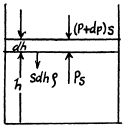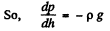(1)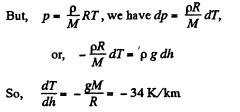That means, temperature of air drops by 34°C at a height of 1 km above bottom.

Q. 14. Suppose the pressure p and the density p of air are related as p/pn = const regardless of height (n is a constant here). Find the corresponding temperature gradient.

Solution. 14.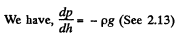(1)

But, from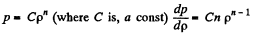(2)

We have from gas low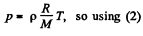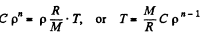Thus,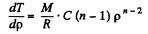But,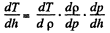so,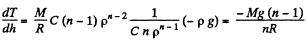Q. 15. Let us assume that air is under standard conditions close to the Earth's surface. Presuming that the temperature and the molar mass of air are independent of height, find the air pressure at the height 5.0 km over the surface and in a mine at the depth 5.0 km below the surface.

Solution. 15. We have, dp = - p g dh and from gas law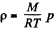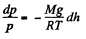Integrating, we get

or,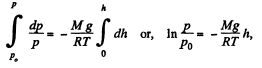(where p0 is the pressure at the surface of the Earth.)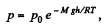[Under standard condition,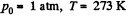Pressure at a height of 5 atm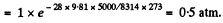Pressure in a mine at a depth of 5 km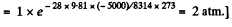Q. 16. Assuming the temperature and the molar mass of air, as well as the free-fall acceleration, to be independent of the height, find the difference in heights at which the air densities at the temperature 0°C differ

(a) e times; (b) by η = 1.0%.

Solution. 16. We have dp = - pg dh but from gas law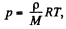Thus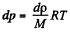at const, temperature

So,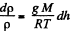Integrating within limits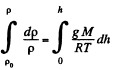or,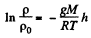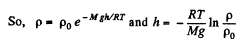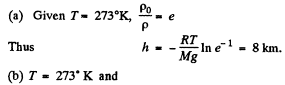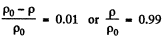Thus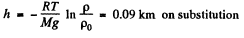Q. 17. An ideal gas of molar mass M is contained in a tall vertical cylindrical vessel whose base area is S and height h. The temperature of the gas is T, its pressure on the bottom base is P0. Assuming the temperature and the free-fall acceleration g to be independent of the height, find the mass of gas in the vessel.

Solution. 17. From the Barometric formula, we have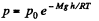and from gas law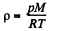So, at constant temperature from these two Eqs.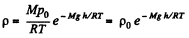(1)

Eq. (1) shows that density varies with height in the same manner as pressure. Let us consider the mass element of the gas contained in the coltimn.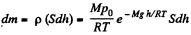Hence the sought mass,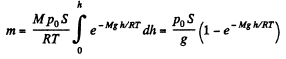Q. 18. An ideal gas of molar mass M is contained in a very tall vertical cylindrical vessel in the uniform gravitational field in which the free-fall acceleration equals g. Assuming the gas temperature to be the same and equal to T, find the height at which the centre of gravity of the gas is located.

Solution. 18. As the gravitational field is constant the centre of gravity and the centre of mass are same. The location of C.M.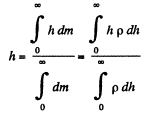But from Barometric formula and gas law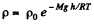So,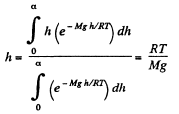Q. 19. An ideal gas of molar mass /If is located in the uniform gravitational field in which the free-fall acceleration is equal to g. Find the gas pressure as a function of height h, if p = p0  at h = 0, and the temperature varies with height as

(a) T = T0  (1 — ah); (b) T = T0  (1 + ah),

where a is a positive constant.

Solution. 19. (a) We know that the variation of pressure with height of a fluid is given by :

dp = - p g dh

But from gas law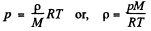From these two Eqs.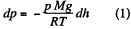or,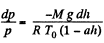Integrating,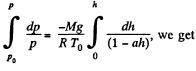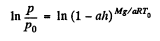Hence,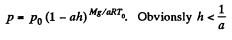(b) Proceed up to Eq. (1) of part (a), and then put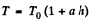and proceed further in the same fashion to get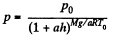Q. 20. A horizontal cylinder closed from one end is rotated with a constant angular velocity ω about a vertical axis passing through the open end of the cylinder. The outside air pressure is equal to P0, the temperature to T, and the molar mass of air to M. Find the air pressure as a function of the distance r from the rotation axis. The molar mass is assumed to be independent of r.

Solution. 20. Let us consider the mass element of the gas (thin layer) in the cylinder at a distance r from its open end as shown in the figure.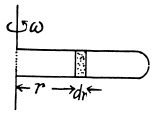Using Newton’s second law for the element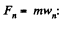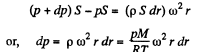So,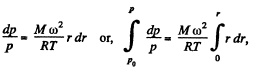Thus,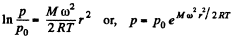Q. 21. Under what pressure will carbon dioxide have the density p = 500 g/1 at the temperature T = 300 K? Carry out the calculations both for an ideal and for a Van der Waals gas.

Solution. 21. For an ideal gas law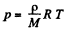So,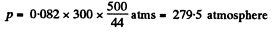For Vander Waal gas Eq.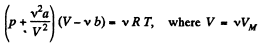or,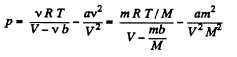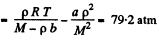Q. 22. One mole of nitrogen is contained in a vessel of volume V = 1.00 1. Find:
(a) the temperature of the nitrogen at which the pressure can be calculated from an ideal gas law with an error η = 10% (as compared with the pressure calculated from the Van der Waals equation of state);
(b) the gas pressure at this temperature.

Solution. 22.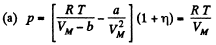(The pressure is less for a Vander Waal gas than for an ideal gas)

or,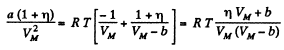or,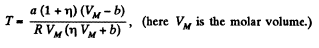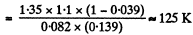(b) The corresponding pressure is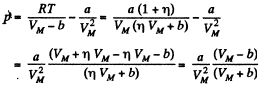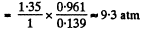Q. 23. One mole of a certain gas is contained in a vessel of volume V = 0.250 1. At a temperature T1 = 300 K the gas pressure is p1 = 90 atm, and at a temperature T= 350 K the pressure is p2 = 110 atm. Find the Van der Waals parameters for this gas.

Solution. 23.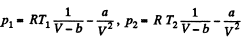So,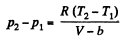or.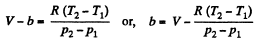Also,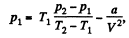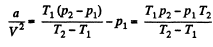or,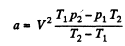Using T1 = 300 K, p1 = 90 atms, T2 = 350 K, p2 = 110 atm, V = 0.250 litre

a = 1.87 atm. litre2/mole2, b = 0.045 litre/mole

Q. 24. Find the isothermal compressibility x of a Van der Waals gas as a function of volume V at temperature T.

Note. By definition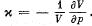Solution. 24.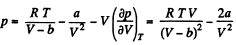or,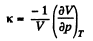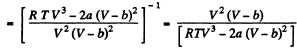Q. 25. Making use of the result obtained in the foregoing problem, find at what temperature the isothermal compressibility x of a Van der Waals gas is greater than that of an ideal gas. Examine the case when the molar volume is much greater than the parameter b.

Solution. 25. For an ideal gas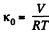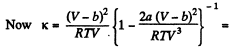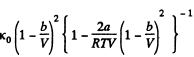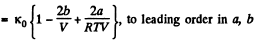Now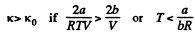If a, b do not vary much with temperature, then the effect at high temperature is clearly determined by b and its effect is repulsive so compressibility is less.

The document Irodov Solutions: Equation of The Gas State Processes - 2 - Notes | Study Physics Class 11 - NEET is a part of the NEET Course Physics Class 11.
All you need of NEET at this link: NEET

## Physics Class 11

127 videos|464 docs|210 tests

## Physics Class 11

127 videos|464 docs|210 tests

### How to Prepare for NEET

Read our guide to prepare for NEET which is created by Toppers & the best Teachers

Track your progress, build streaks, highlight & save important lessons and more!

,

,

,

,

,

,

,

,

,

,

,

,

,

,

,

,

,

,

,

,

,

;